# Chocolate

Chocolate, which originally cost 1.5 euros, was increased by 40%. How many euros did it cost?

x =  2.1 Eur

### Step-by-step explanation: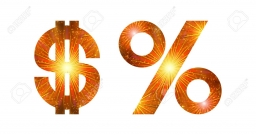Did you find an error or inaccuracy? Feel free to write us. Thank you!Tips to related online calculators

## Related math problems and questions:

• The notebook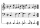After rising by 40%, the notebook cost 10.50 euros. How much did this notebook cost if it increased in price by only 20% instead of 40%?
• Bike cost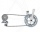The father gave his son € 100 to buy a bicycle, which was 40% of the total amount of the bicycle. How much did the bike cost?
• SkisSkis, which cost € 800, became 25% more expensive before the season. After the season, they became cheaper again by 25%. How many euros did the skis cost after the discount?
• Rising priceThe book first become more expensive by 5 euros. New price was later increased by 8%. After the second price increase book cost 46 euros. What was the original price of the book?
• Tennis racketTennis racket became cheaper by 16% in winter. In the spring, the price increased by 15%. After this increase, it cost € 38. How much did the rocket cost in the winter before cheapening?
• The blueThe blue and red coats originally cost the same. Then red prices rose by 27% and blue prices fell by 87%. Then their price differed by CZK 300. How much did the originally blue coat cost?
• Washing machineThe price of washing machine was decreased by 21% and then by 55 € due small sales. After two price decreases cost € 343. How much was originally cost?
• Budget planIn the construction of the building, the planned budget exceeded 13%, which was 32,500 euros. How many euros cost built the building?
• Product V2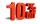The price of product was increased by 12%. Price increased by 273. How much money it would cost this product, if it was on the contrary by 19% discounted.
• Savings and retirement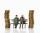The older sibling has saved 44% more euros than the youngest, which represents 22 euros. How many euros older brother has saved ?
• MerchantThe merchant lower cost by 10% in December when it was not sold. Again in January lower cost by 20% and now costs 576 €. A. How much did the goods stand originally? B, how much cost the goods after the first lowering? C, How many percents total merchant l
• Test pointsIf you earned 80% of the possible 40 points, how many points did you miss to get 100%?
• Sales off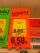shoes cost x euros. First they are discounted by 50%, and then 25% of the new amount. Determine what percentage of the total cost is cheaper.
• Price inflationThis year the average wage grew by 4.5%. Prices rose by 2%. How much did the real wage increase in the given year?
• Parcel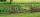Both dimensions of the rectangular parcel were increased by 31%. By how many % has increased its acreage?
• Ice skates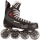Ice skates were raised twice, the first time by 25%, the second time by 10%. After the second price, their cost was 82.5 euros. What was the original price of skates?
• Profit margin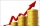If total sales for the month are \$450,000 and the profit margin is 40%, how much was the cost of goods sold?